# Eigenvalues and Eigenvectors of 3×3 Matrix Example

Find the eigenvectors and eigenvalues of the following matrix: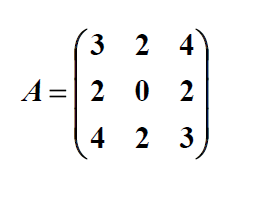Solution:

To find eigenvectors we must solve the equation below for each eigenvalue: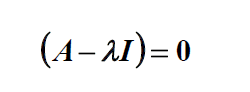The eigenvalues are the roots of the characteristic equation: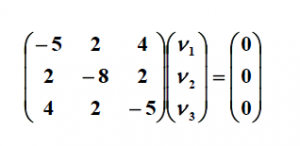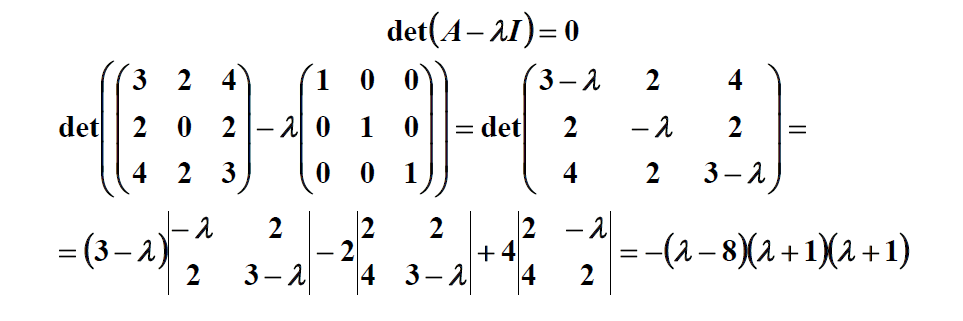The solutions of the equation above are eigenvalues and they are equal to: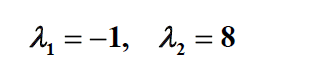Eigenvectors for: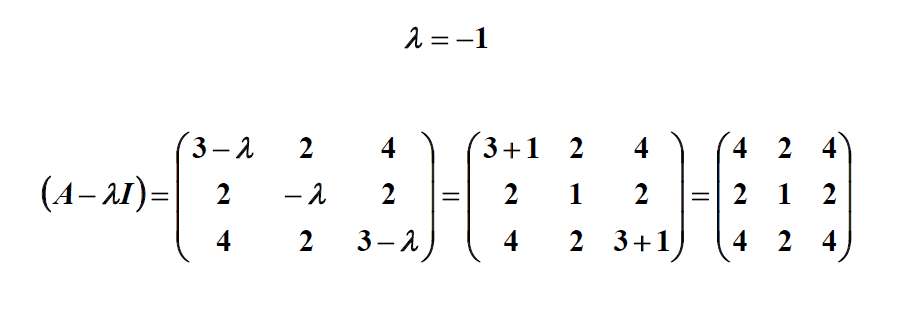Now we must solve the following equation: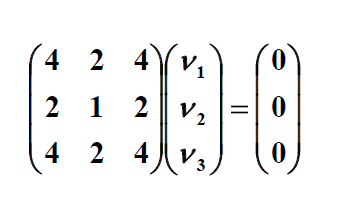First let’s reduce the matrix: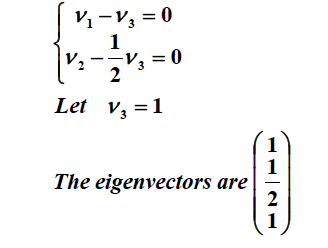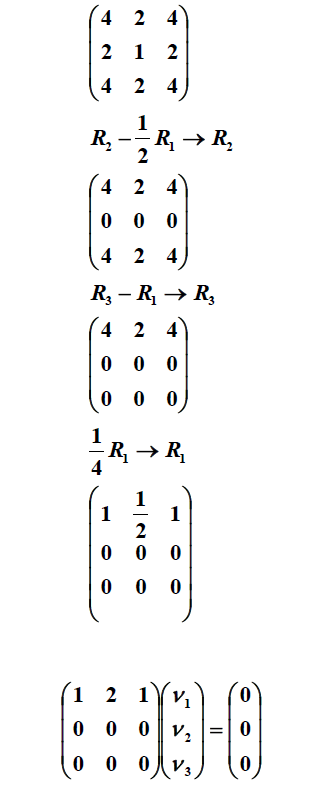This reduces to the equation: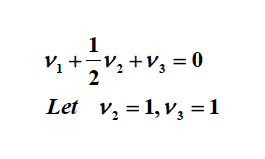Eigenvectors for: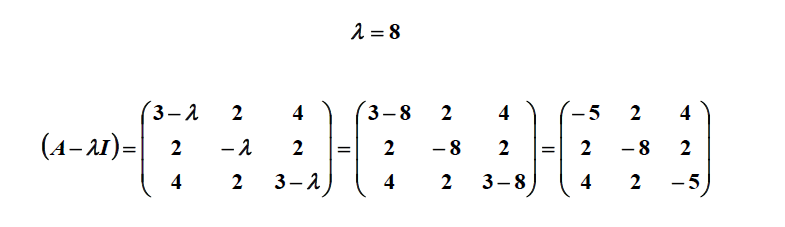Now we must solve the following equation: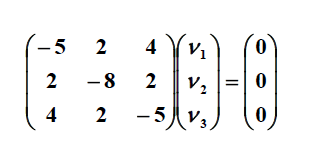First let’s reduce the matrix: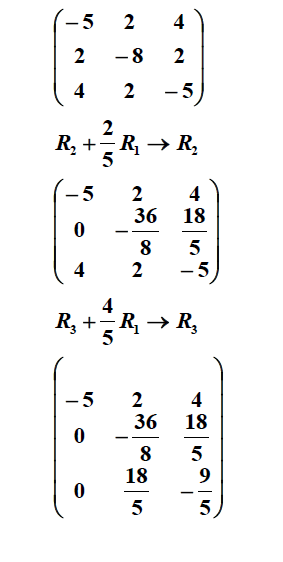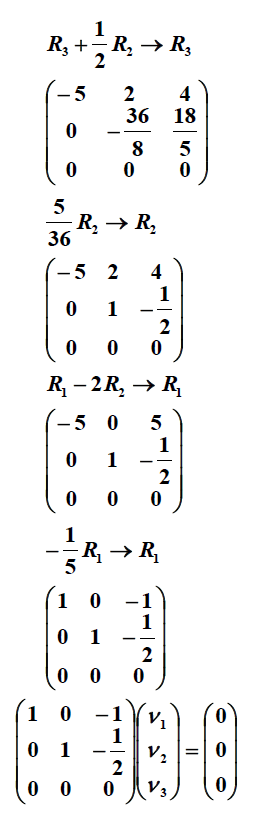This reduces to the equation:There are two kinds of students: those who love math and those who hate it. If you love it, our example of the solution to eigenvalues and eigenvectors of 3×3 matrix will help you get a better understanding of it. This example was made by one of our experts; you can easily contact them if you are puzzled with complex tasks in math.

If you have trouble understanding your eigenvalues and eigenvectors of 3×3 matrix assignment, there is no need to panic! Get professional help with your math assignment at any time that is convenient for you. We have gathered a team of experts in math who can easily solve even the most difficult math assignments. Improve your math skills with us!

AssignmentShark works day and night to provide expert help with assignments for students from all over the world. When you need prompt help, ask our professionals, as they are able to complete your assignment before the deadline. Also, to make our service affordable, we have provided reasonable prices so every student can afford our services. There is no time to wait for assistance! Get assignment help online done with our experts!